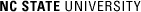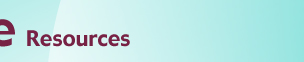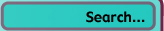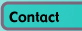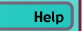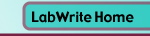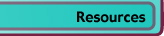# Graphing Resources

## Representing Significant Digits

### Introduction

You've collected ten measurements on the distance a marble rolled in a Physics lab. You calculate the mean distance and want to record this value in your lab. Do you record it as:

112.3895 or
112.3 or
112?

This page will give you some guidance on how to report your experimental results with the appropriate number of significant digits.

When documenting experimental data, it is important not to state the data with too much precision. That is, the number of significant digits should reflect the level of experimental uncertainty. How do you know what the level of experimental uncertainty and, therefore, the precision of your measurements is? The best way is to take multiple measurements and perform some basic statistical calculations. Short of that, you can also make visual inspections of both the instrumentation you are using and the data you have collected. First we will look at visual inspection methods and then the statistical calculations.

### Visual Inspection of Equipment

If you are recording data using analog equipment, then you will typically be reading values from a dial, gauge, or other scale that represents tick marks for the major or minor units. What are the major or minor units on the scale? Is the space between the smallest tick marks small enough that you can interpolate a fractional distance between two marks (e.g., the pointer is one quarter the way over to the next mark)? The smallest unit (or fraction of a unit) you can read should give you an idea what the precision of your measurement is. You should then use this as a guide for reporting any values derived from subsequent calculations. For example, lets say that youve taken six measurements that can be read to the nearest millimeter:

11, 12, 15, 14, 13, 14

It would not be appropriate to report the mean to three significant digits: 13.167. Instead, you would probably want to use, at most, the tenths digit: 13.2.

Visually inspecting the range of values in your data set will also help you decide the number of significant digits to display. For example, youve taken six measurements in centimeters:

12, 45, 89, 36, 22, 53

Given that the data is ranging not just in the singles digit, but also in the tens digit (from 12 to 89), you would want to report the mean as 43, not as 42.8.

When taking readings using digital equipment, the readout will often give an unjustified confidence in the precision of your readings. Say, for instance, you take repeated measurements of voltages on a digital voltmeter:

2.3483, 3.2958, 1.8934, 5.3279, 4.4423

This is an unreasonable display of precision given your values range between 1.9 and 5.3. While it is possible the voltmeter can read to this level of precision, in theory, your experimental setup is not allowing for this level of precision. A better representation of this data would be:

2.3, 3.3, 1.9, 5.3, 4.4

### Statistical Calculations

With some simple statistical calculations, you can come up with a much better representation of the uncertainty in your data. In addition to calculating the mean (i.e., average) of a set of measurements, you can also calculate the standard deviation of the measurements. The standard deviation (S.D.) characterizes the average uncertainty of the measurements. Operationally, if you continued to take measurements, approximately 68% of your measurements would fall within a distance of one S.D. on either side of the mean of these measurements. If we take the first data set above:

11, 12, 15, 14, 13, 14

If we continued to take measurements, we could be pretty confident that 68% of the measurements would fall within:

13.2 +/- 1.5 mm

Here, the uncertainty is represented by the S.D.

When reporting the mean, it is often preferred to give the standard deviation of the mean, or standard error. The standard error (S.E.) reflects the number of measurements that are taken and grows smaller as the number of measurements (N) grows larger. For the same data set above, the uncertainty represented by the S.E. would be:

13.2 +/- 0.6 mm

Now, getting back to significant digits. The general rule is that experimental uncertainties should be rounded to one significant digit. All of the following are appropriate notations:

13.2 +/- 0.6 mm

43 +/- 11 cm

8.76 +/- 0.02 volts

It follows that the reported value of the mean (or other value) should be of the same order of magnitude as the experimental uncertainty.

For more information on statistical calculations and graphical display of uncertainty, see the graphing tutorial on Using Error Bars in Graphs.

### Reference

Taylor, J. R. (1997). Error analysis: The study of uncertainties in physical measurements (2nd ed.). Sausalito, CA: University Science Books.© Copyright NC State University 2004 Sponsored and funded by National Science Foundation (DUE-9950405 and DUE-0231086) Site design by Rosa Wallace Rev. RW 5/16/05